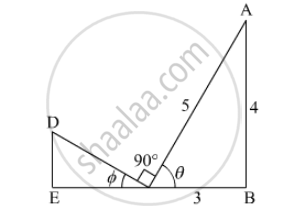Advertisement Remove all ads

# In the Following Figure the Value of Cos ϕ is - Mathematics

MCQ

In the following figure  the value of cos ϕ is#### Options

• $\frac{5}{4}$

• $\frac{5}{3}$

• $\frac{3}{5}$

• $\frac{4}{5}$

Advertisement Remove all ads

#### Solution

We should proceed with the fact that sum of angles on one side of a straight line is180°.

So from the given figure,

θ+∅+90°=180° 

So , θ=90°-∅.............(1) 

Now from the triangle ΔABC,

sin ∅=4/5

Now we will use equation (1) in the above,

sin(90°-∅)=4/5

Therefore, cos∅=4/5`

Is there an error in this question or solution?
Advertisement Remove all ads

#### APPEARS IN

RD Sharma Class 10 Maths
Chapter 10 Trigonometric Ratios
Q 34 | Page 59
Advertisement Remove all ads
Advertisement Remove all ads
Share
Notifications

View all notifications

Forgot password?